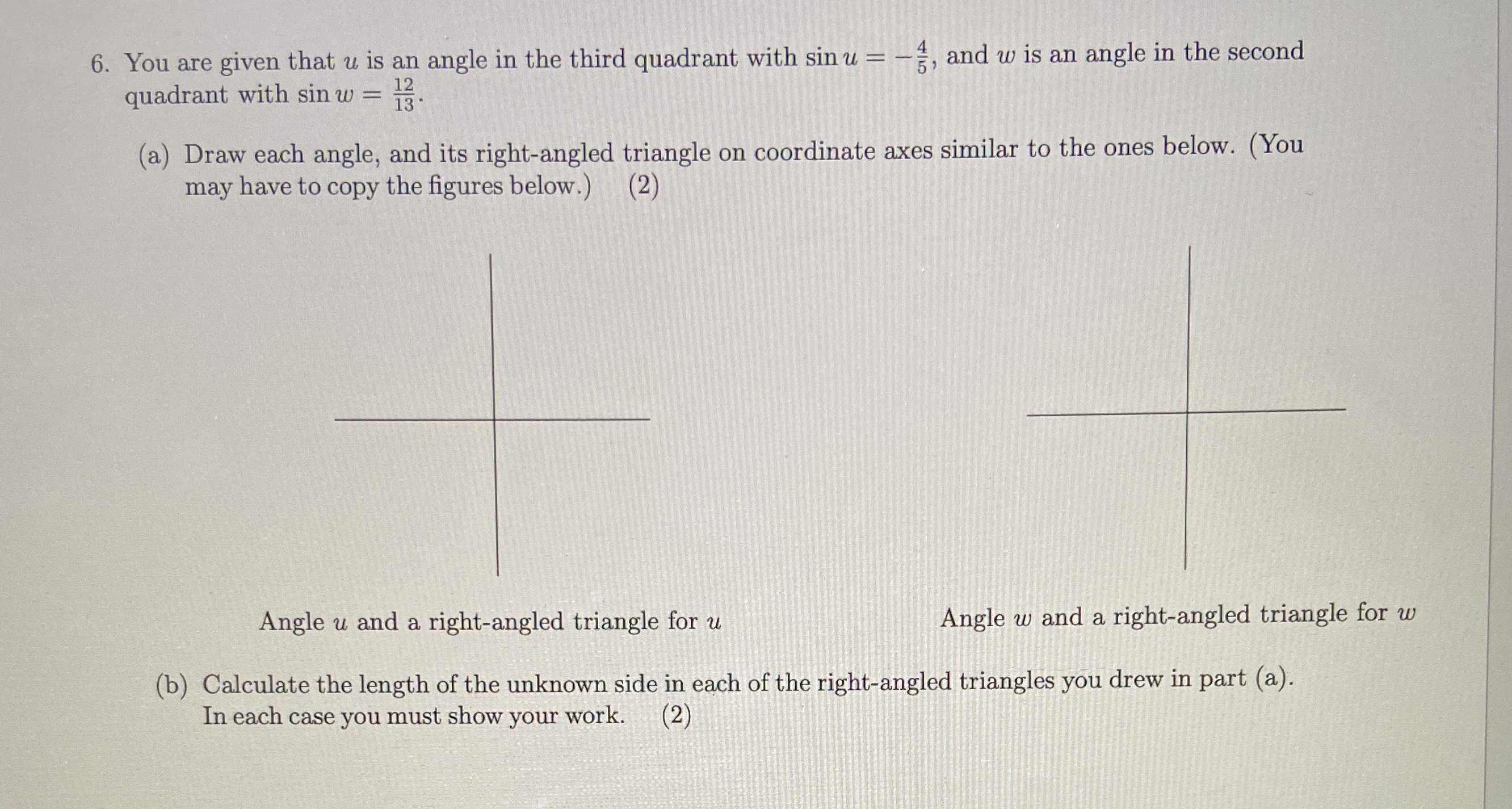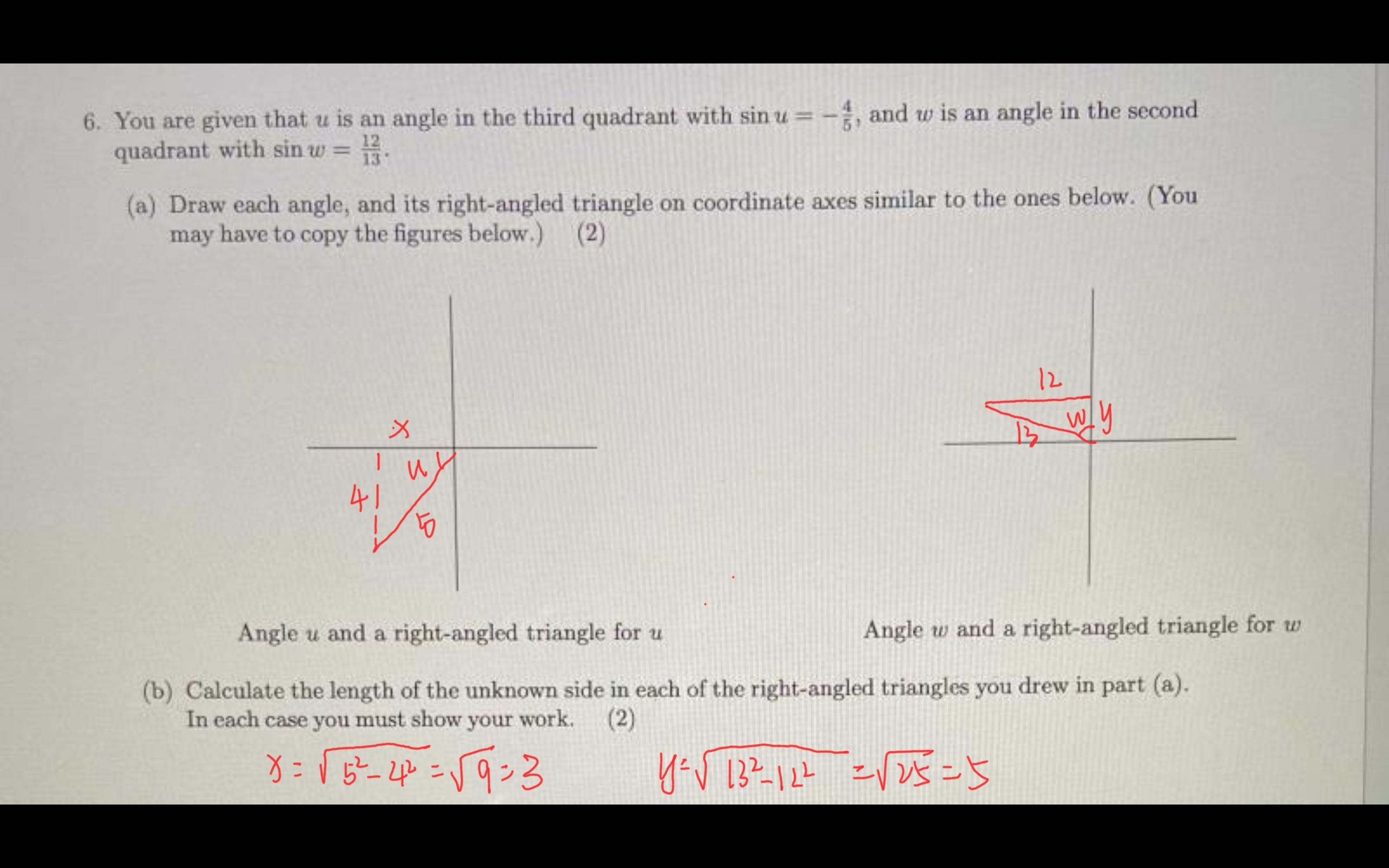### ¿Todavía tienes preguntas de matemáticas?

Pregunte a nuestros tutores expertos
Algebra
Pregunta6. You are given that $$u$$ is an angle in the third quadrant with $$\sin u = - \frac { 4 } { 5 }$$ , and $$w$$ is an angle in the second quadrant with $$\sin w = \frac { 12 } { 13 }$$ .

(a) Draw each angle, and its right-angled triangle on coordinate axes similar to the ones below. (You may have to copy the figures below.) (2)

(b) Calculate the length of the unknown side in each of the right-angled triangles you drew in part (a). In each case you must show your work. (2)